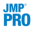Publication date: 04/12/2021

## Validation Methods for Neural

Neural utilizes a validation method in its fitting routine. Choices of validation method include holdback, K-fold, or (in JMP Pro) the use of a validation column. To fit a model, the Neural platform does the following:

applies a penalty on the model parameters

uses the validation set to tune the penalties on the parameters.

Select one of the following validation methods:

Excluded Rows Holdback

Uses row states to subset the data. Rows that are unexcluded are used as the training set, and excluded rows are used as the validation set.

For more information about using row states and how to exclude rows, see Hide and Exclude Rows in Data Tables in Using JMP.

Holdback

Randomly divides the original data into the training and validation sets. You specify the proportion of the original data to use as the validation set (holdback). The random selection is based on stratified sampling across the model factors to attempt to create training and validation sets that are more balanced than ones based on simple random sampling.

KFold

Divides the data into K subsets, or folds. In turn, each of the K folds is used to validate the model fit on the rest of the data, fitting a total of K models. The final model is for the fold that resulted in the model with the best validation statistic.

This method is useful for small data sets, because it makes efficient use of limited amounts of data.Validation Column

Uses a numeric column that defines the validation sets. The column’s values determine how the data is split:

If the validation column has two levels, the smaller value defines the training set and the larger value defines the validation set.

If the validation column has three levels, the values, in order of increasing size, define the training, validation, and test sets.

If the validation column has more than three unique values, then K-Fold validation is performed. The number of folds is determined by the number of values in the validation column.

The Neural platform uses the validation column to train and tune the model or to train, tune, and evaluate the model. For more information about validation, see Validation in JMP Modeling.

Want more information? Have questions? Get answers in the JMP User Community (community.jmp.com).